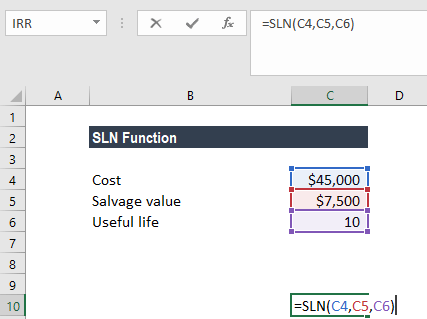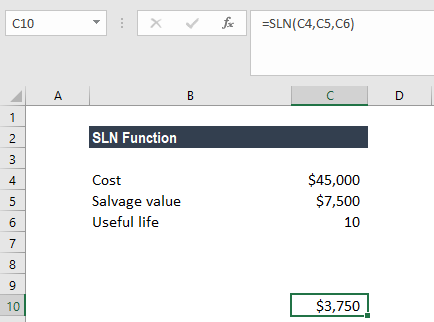# SLN Function

Calculate the depreciation of an asset on a straight-line basis

## What is the SLN Function (Straight Line)?

The SLN function will calculate the depreciation of an asset on a straight-line basis for one period. In financial modeling, the SLN function helps calculate the straight line depreciation of a fixed asset when building a budget.

### Formula

=SLN(cost, salvage, life)

The SLN function uses the following arguments:

1. Cost (required argument) – This is the initial cost of the asset.
2. Salvage (required argument) – The value at the end of the depreciation (sometimes called the salvage value of the asset).
3. Life (required argument) – This is the number of periods over which the asset is depreciated (sometimes called the useful life of the asset).

#### Notes

• Straight-line depreciation is the simplest way of calculating the depreciation of a fixed asset.
• The formula used in calculating straight-line depreciation is:Where:

• Cost is the initial cost of the asset (at the start of period 1).
• Salvage is the final value of the asset at the end of its lifetime.

The straight-line depreciation method subtracts a fixed amount from the value of an asset during each period of the asset’s lifetime.

### How to use the SLN Depreciation Function in Excel?

To understand the uses of the SLN function, let’s consider an example:

#### Example – Straight-line depreciation

Suppose we are given the following data and we need to calculate the depreciation using the straight-line method:

• Cost of the asset: \$45,000
• Salvage value (if any): \$7,500
• Useful life (in years): 10

To calculate the straight-line depreciation, we will use the following formula:We get the result below:\$3,750 would be the depreciation to charged yearly for the given asset.

If the asset was purchased in the middle of the year after the start of the financial year, the depreciation would be charged on a pro-rated basis.

### A few notes about the SLN Depreciation Function

1. #DIV/0! error – Occurs if the given life argument is equal to zero.
2. #VALUE! error – Occurs if any of the given argument is non-numeric.

Thanks for reading CFI’s guide to important Excel functions! By taking the time to learn and master these functions, you’ll significantly speed up your financial modeling & analysis. To learn more, check out these additional CFI resources:

• Excel Finance Functions